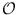Next: Dijkstra(flexible) ( GIT_DIJKSTRA ) Up: Graphs and Iterators Previous: Topological Sort (flexible) (   Contents   Index

# Strongly Connected Components (flexible) ( GIT_SCC )

Definition

An instance algorithm of class GIT_SCC< Out, In, It, OutSt, InSt, NSt, Mark > is an implementation of an algorithm that computes the strongly connected components.

Iterator version: There is an iterator version of this algorithm: SCC_It. Usage is similar to that of node iterators without the ability to go backward in the sequence and only a graph is allowed at creation time. Method compnumb() returns the component number of the current node.

#include < LEDA/graph/graph_iterator.h >

Creation

 GIT_SCC< Out, In, It, OutSt, InSt, NSt, Mark > algorithm(OutSt ost, InSt ist, Mark ma, Out oai, const It& it, In iai) creates an instance algorithm of this class bound to the stack st and data accessor ma.

Preconditions:

• Out is an adjacency iterator that iterates over the outgoing edges of a fixed vertex
• In is an adjacency iterator that iterates over the incoming edges of a fixed vertex
• OutSt is stack parameterized with items of type Out
• InSt is stack parameterized with items of type In
• Mark is a data accessor that has access to a boolean value that is associated with each node of the graph

Operations

 int algorithm.state() returns the internal state.

• NEXT_OUT =first phase,
• NEXT_ORDER =order phase,
• NEXT_IN=second phase,
• NEXT_DONE =algorithm finished

 void algorithm.finish_algo() executes the algorithm until finished() is true. bool algorithm.finished() returns true if the algorithm is finished. InSt& algorithm.get_in_stack() gives direct access to the internal stack of incoming adjacency iterators. In algorithm.in_current() returns the current iterator of the internal stack of incoming adjacency iterators. OutSt& algorithm.get_out_stack() gives direct access to the internal stack of outgoing adjacency iterators. Out algorithm.out_current() returns the current iterator of the internal stack of outgoing adjacency iterators. itnodetype algorithm.current_node() returns the current node. int algorithm.compnumb() returns the component number of the fixed node of the current iterator if current state is NEXT_IN. int algorithm.next() Performs one iteration of the core loop of the algorithm.

Implementation

Each operation requires constant time. The algorithm has running time(| V| + | E|).Next: Dijkstra(flexible) ( GIT_DIJKSTRA ) Up: Graphs and Iterators Previous: Topological Sort (flexible) (   Contents   Index